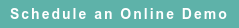# Blog

### Posts by Topic

The U.S. transfer pricing regulations prescribe under 26 CFR 1.482-1(e)(2)(iii)(B): “The interquartile range [IQR] ordinarily provides an acceptable measure of this [arm’s length] range; however[,] a different statistical method may be applied if it provides a more reliable measure.”

The U.S. transfer pricing regulations refer to “most reliable” or “more reliable” -- which means (following the statistical principle of minimum variance) the narrowest range computed from the dataset. See Wonnacott (1969), Chapter 7-2 (Desirable properties of estimators), pp. 134-139.

Regression analysis is the accepted method to estimate the parameters of a statistical relationship between two or more variables. Under 26 CFR 1.482-5(b)(4), the U.S. regulations define profit indicators as the ratio between operating profits on the numerator and varying denominators, such as net sales (revenue), total cost and expenses, or operating assets. In practice, regression analysis may provide a more reliable statistical range of the slope coefficient of a linear operating profit equation than IQR.

Instead of using accounting ratios to compute quartiles, I let the numerator (Y) be the dependent variable, and the denominator (X) be the independent variable. Next, I specify the unknown proportionality parameter (slope coefficient = profit indicator) to be determined in a linear regression equation:

(1) Y = β X + U

where U represents uncertainty because the relationship between X and Y is not exact.

The intercept is assumed = 0, which is a testable hypothesis. Experiments in “exact” sciences, such as physics, are also subject to random errors. See Cowan (1998).

In regression eq. (1), the independent variable X is an N by 2 matrix, and the dependent variable Y is a column vector with N rows. The row count N is the number of observations in the dataset.

From the central limit theorem (law of large numbers), as N increases the estimated coefficients and standard errors become more reliable. See Wonnacott (1969), pp. 112-113, 241, Kemeny, Snell & Thompson (1974), pp. 277-281, or Cowan (1998), pp. 33, 147. Loève (1963) covers the convergent law of large numbers ad nauseam.

After taking the expected value (E) of eq. (1), the uncertainty vanishes. This means that on the average, the variable U has a null effect determining Y:

(2) E(Y) = β E(X) because E(U) = 0.

The regression estimate of the slope parameter β is computed using the formula:

(3) b = SUM(X – Mean(X))(Y – Mean(Y)) / SUM(X – Mean(X))2

See Draper & Smith (1966), eq. 1.2.9, p. 10. The estimated parameter “b” for beta is subject to errors, such that a statistical interval (range) around “b” can be calculated with the formula:

(4) b ± SE(b)

The symbol SE denotes the standard error. The standard error of the slope coefficient “b” in eq. (1) can be computed with the formula:

(5) SE(b) = λ × [SE(U) / SE(X)]

See Draper & Smith (1966), eq. 1.4.2, p. 19. The displacement parameter λ = 1 / SQRT(Count – 2) and Count is the number of observations in the comparables dataset. See also Hahn & Meeker (1991).

The “point estimate” of the slope coefficient “b” is a measure of the data central value, but it provides no information about its reliability. Thus, it’s necessary to compute a statistical interval around the point estimate to determine its precision or reliability. In this regard, it’s important to apply the statistical principle of minimum variance and select the model that provides the most reliable measure of the point estimate.

The formulae above are available in statistics textbooks (see references below). Online statistics packages, such as Econometrica, can be used to estimate “b” and the applicable standard errors. The regression algorithms are also available online in RoyaltyStat.

The Gauss-Markov theorem proves that among linear unbiased estimators of the intercept and slope coefficients, the least squares estimators of regression analysis have minimum variance. See Draper & Smith (1966), pp. 59-60, Wonnacott (1969), pp. 240-241, or Weisberg (1985), p. 14.

References

Glen Cowan, Statistical Data Analysis, Oxford University Press, 1998.

Norman Draper & Harry Smith, Applied Regression Analysis, Wiley, 1966 (3rd edition is available).

Gerald Hahn & William Meeker, Statistical Intervals, John Wiley, 1991.

Michel Loève, Probability Theory (3rd edition), Nostrand, 1963.

John Kemeny, Laurie Snell & Gerald Thompson, Introduction to Finite Mathematics (3rd edition), Prentice-Hall, 1974.

Stephen Stigler, History of Statistical Concepts and Methods, Harvard University Press, 1999, p. 320: “The method of least squares is the automobile [engine] of modern statistical analysis.”

Sanford Weisberg, Applied Linear Regression (2n edition), John Wiley, 1985.

Thomas Wonnacott & Ronald Wonnacott, Introductory Statistics, John Wiley, 1969 (3rd edition is available, but I prefer the first edition cited here).

Published on Dec 19, 2022 12:16:47 PM

Ednaldo Silva (Ph.D.) is founder and managing director of RoyaltyStat. He helped draft the US transfer pricing regulations and developed the comparable profits method called TNNM by the OECD. He can be contacted at: esilva@royaltystat.com

RoyaltyStat provides premier online databases of royalty rates extracted from unredacted license agreements
and normalized company financials (income statement, balance sheet, cash flow). We provide high-quality data, built-in analytical tools, customer training and attentive technical support.